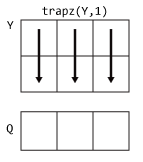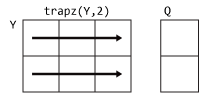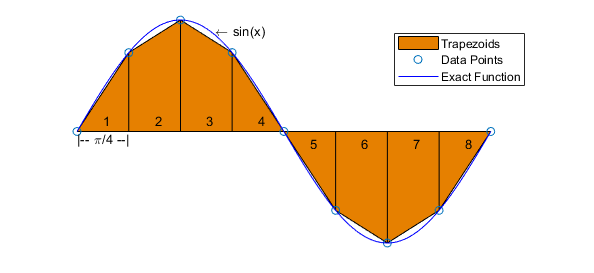# trapz

## 语法

``Q = trapz(Y)``
``Q = trapz(X,Y)``
``Q = trapz(___,dim)``

## 说明

``Q = trapz(Y)` 通过梯形法计算 `Y` 的近似积分（采用单位间距）。`Y` 的大小确定求积分所沿用的维度：如果 `Y` 为向量，则 `trapz(Y)` 是 `Y` 的近似积分。如果 `Y` 为矩阵，则 `trapz(Y)` 对每列求积分并返回积分值的行向量。如果 `Y` 是多维数组，则 `trapz(Y)` 对大小不等于 1 的第一个维度求积分。该维度的大小变为 1，而其他维度的大小保持不变。`

````Q = trapz(X,Y)` 根据 `X` 指定的坐标或标量间距对 `Y` 进行积分。 如果 `X` 是坐标向量，则 `length(X)` 必须等于 `Y` 的大小不等于 1 的第一个维度的大小。如果 `X` 是标量间距，则 `trapz(X,Y)` 等于 `X*trapz(Y)`。 ```

``Q = trapz(___,dim)` 使用上述任意语法沿维度 `dim` 求积分。必须指定 `Y`，也可以指定 `X`。如果指定 `X`，则它可以是长度等于 `size(Y,dim)` 的标量或向量。例如，如果 `Y` 为矩阵，则 `trapz(X,Y,2)` 对 `Y` 的每行求积分。`

## 示例

`Y = [1 4 9 16 25];`

`Y` 包含域 [1, 5] 中的 $f\left(x\right)={x}^{2}$ 的函数值。

`Q = trapz(Y)`
```Q = 42 ```

`X = 0:pi/100:pi;`

`Y = sin(X);`

`Q = trapz(X,Y)`
```Q = 1.9998 ```

```X = [1 2.5 7 10]; Y = [5.2 7.7 9.6 13.2; 4.8 7.0 10.5 14.5; 4.9 6.5 10.2 13.8];```

`Q1 = trapz(X,Y,2)`
```Q1 = 3×1 82.8000 85.7250 82.1250 ```

```x = -3:.1:3; y = -5:.1:5; [X,Y] = meshgrid(x,y);```

`F = X.^2 + Y.^2;`

`trapz` 对数值数据、而不是函数表达式求积分，因此表达式通常无需已知可对数据矩阵使用 `trapz`。在已知函数表达式的情况下，您可以改用 `integral``integral2``integral3`

`$I={\int }_{-5}^{5}{\int }_{-3}^{3}\left({x}^{2}+{y}^{2}\right)dx\phantom{\rule{0.2222222222222222em}{0ex}}dy$`

`I = trapz(y,trapz(x,F,2))`
```I = 680.2000 ```

`trapz` 先对 x 求积分以生成列向量。然后，y 上的积分可将列向量减少为单个标量。`trapz` 稍微高估计确切答案 680，因为 f(x,y) 是向上凹的。

## 输入参数

• 如果 `X` 是标量，则它指定数据点之间的间距为均匀间距，并且 `trapz(X,Y)` 等于 `X*trapz(Y)`

• 如果 `X` 是向量，则它指定数据点的 x 坐标，并且 `length(X)` 必须与 `Y` 的积分维度大小相同。

• `trapz(Y,1)``Y` 的列中的连续元素进行处理并返回行向量。• `trapz(Y,2)``Y` 的行中的连续元素进行处理并返回列向量。## 详细信息

### 梯形法

`trapz` 通过梯形法执行数值积分运算。通过将一个区域分为包含多个更容易计算的区域的梯形，该方法对区间内的积分计算近似值。例如，下面是使用八个均匀间隔的梯形对正弦函数求梯形积分：`$\begin{array}{c}\underset{a}{\overset{b}{\int }}f\left(x\right)dx\text{\hspace{0.17em}}\text{\hspace{0.17em}}\approx \text{\hspace{0.17em}}\text{\hspace{0.17em}}\frac{b-a}{2N}\sum _{n=1}^{N}\left(f\left({x}_{n}\right)+f\left({x}_{n+1}\right)\right)\\ =\frac{b-a}{2N}\left[f\left({x}_{1}\right)+2f\left({x}_{2}\right)+...+2f\left({x}_{N}\right)+f\left({x}_{N+1}\right)\right]\text{\hspace{0.17em}}\text{\hspace{0.17em}},\end{array}$`

`$\underset{a}{\overset{b}{\int }}f\left(x\right)dx\text{\hspace{0.17em}}\text{\hspace{0.17em}}\approx \text{\hspace{0.17em}}\text{\hspace{0.17em}}\text{\hspace{0.17em}}\frac{1}{2}\sum _{n=1}^{N}\left({x}_{n+1}-{x}_{n}\right)\left[f\left({x}_{n}\right)+f\left({x}_{n+1}\right)\right]\text{\hspace{0.17em}},$`

## 提示

• 使用 `trapz``cumtrapz` 对离散数据集执行数值积分。如果数据可以用函数表达式表示，则改用 `integral``integral2``integral3`

• `trapz` 将它执行运算的维度大小减小到 1，并仅返回最终的积分值。`cumtrapz` 还返回中间积分值，保留它执行运算的维度的大小。